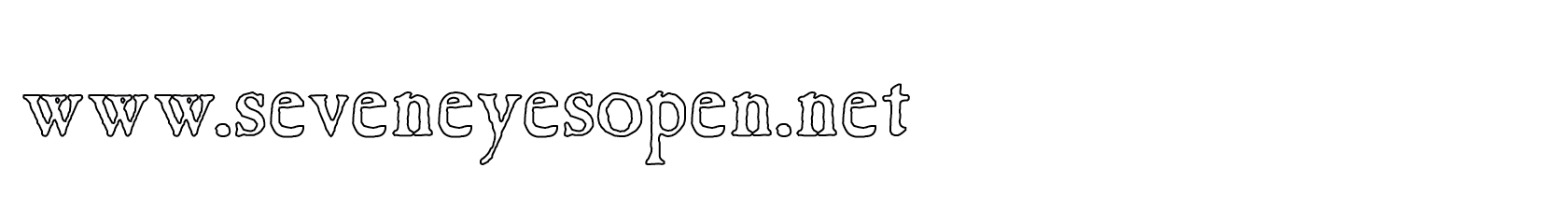None:
Polyps:
Strongs:MetaphysicsGiven All The Facts In every subgroup containing virtue it is simple enough to state that the conjunctions of r and s etc. are empty and not in the indexing set. But with the four subgroups that do not so contain virtue; does the indiscernibility of positive properties from a virtue suffice to close the sets? I argue that yes, it most certainly does. Each of the six properties not virtues are constructed in the maximal sense as in predicates of sets; being all "a" such that a=>r or all "b" such that r=>b etc. In these properties it is easy enough to argue that these equivalents are "indiscernible enough" so that the statement that for instance, given v, I have the K4 triple r + u&v = v. I must argue that r&u&v is not in the indexing set. Now in the book it is argued that r and u&v are disjoint sets. Then the intersection is empty. That is not to state that r&u&v is not a positive property, is it? If Pos(r&u&v) then N¬(Pos(r&u&v)-1) <=> N¬(r-1&¬(u&v)) which is also to state that; r-1=>u&v or (u&v)-1=>r. Then the positivity of the excluded middle implies that the disjunction r v (u&v) holds, but then there is no excluded middle. So the inconsistency of Pos(r&u&v) is shown. it may not be a positive property: it privates itself. (There is also no equivalence to virtue, one property must be axiomatically "positive". The statement should be properly formed: I.e. r v (u&r-1=> u&v). Then N¬(u&r-1&¬v) or N(r&v&¬u) and Pos(r&u&v) is "impossible".) Likewise given Pos(x&y) I have "x v y" in every sense it may be applied: The facts are such that it simply holds that each triple from the octal which together form subgroups are also closed. Then what may I state of Pos(x&y)-1? only that Pos(x-1&y-1) holds and so x and y hold as negative. Yet once again this follows from the disjunction on both sides. Then because x&y is not a positive property, (x&y)-1 is not exemplifiable in a positive sense as given the same disjunction, (x&y)-1 is or must be "out of scope". I should limit the indexing set again. In fact given p, the 'negation' of (r&s)-1 is not r&s but is actually u&v. If I am limited to the indexing set {p, r, s} and r&s is certainly not in the filter then it does not follow its complement must be! This complement would be in I \ W for some W in U, so must be in the indexing set: but I modified the indexing set to exclude r&s and its inverse! There is no quandary! Then x&y is not positive, and may never be so, then what of its negation (x&y)-1? In fact, all I may state is that given free exercise between x and y, x&y is negative. (may never be positive.) There is one fallacy of equivocation to watch for and that is for (x&y)-1 to be mistaken for a virtue. (But it is entailed from both sides of r and s). So then, every triple forming a K4 subgroup of the octal has a different indexing set: one that does not contain whatever analogue of x&y and (x&y)-1 is not "in scope" (in the indexing set) and also the union of these indexing sets is the whole set of positive properties - the ultrafilter of the octal group itself. It would almost seem that a lack of free exercise between Pos((x&y)-1) and Pos(x&y) is enough to exclude (x&y)-1 from the indexing set. Continue To Next Page Return To Section Start Return To Previous Page'